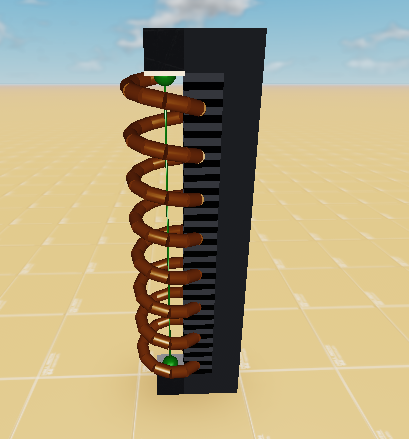# How do you put real units to spring constraints?... Or is it possible to do so?

I’m working on putting actual units on spring constraints like lbs/in and kg/mm and I got a good start thanks to this devforum post and how A-Chassis calculates weight.

I made this model with a 5 stud ruler and a fixed spring right next to it, the ruler represents 50 inches since I’m following 1 stud = 10-inch on this one.
Spring model.rbxm (6.8 KB)The model is a total of 6 studs in height if you include the 0.5-stud cubes that hold the spring constraint and I made the following script for the spring to put real units on the spring’s stiffness.

``````local simulatorModel = script.Parent.SpringSimulator
local weightBrick 	 = simulatorModel.WeightBrick

--[[Variables]]--
local simulatorGravity = 35
local brickWeight 	   = 5 --lbs
--Brick
weightBrick.Size       = Vector3.new(0.5,0.5,0.5)
--Spring
local springRate  	   = 5 --lbs/in
local springDamping    = 1
local springLength     = 5

--[[calculations & stuff]]--
local densityCalc 	 = (brickWeight/2.205/8)/(weightBrick.Size.x*weightBrick.Size.y*weightBrick.Size.z*1000/61024)/1000
weightBrick.CustomPhysicalProperties = PhysicalProperties.new(densityCalc,0,0,0,0)

local springConstraint = simulatorModel.Spring
springConstraint.Stiffness  = springRate*1.25
springConstraint.Damping    = springDamping
springConstraint.FreeLength = springLength

local bForce = Instance.new('BodyForce', weightBrick)
bForce.Name  = 'Gravity Force'
bForce.Force = Vector3.new(0, weightBrick:GetMass()*(workspace.Gravity-simulatorGravity), 0)
``````

From the script, we got a 5 lbs 0.5 stud brick and a spring rate of 5 lbs/in. When you run the model, nothing went wrong, but as soon as you resize the brick to whatever, that’s when you’ll see the problem. The size of the brick can affect the weight even without the current weight calculation as the one in the script. I already have an idea of how to fix it, but I just don’t know how to implement it. I know that raycasting would be the best choice for putting real units to springs and stuff, but I would also like to see if it’s possible to use real units on spring constraints.

Units of measurements are relative to the human body and history. To standardize Roblox to the real perception of the SI system, you’d have to measure the average height of the Roblox character.

Let’s use the average height of a human male: 5 ft. 9 in., or approx. 1.75 meters.

Based on the average height of a human male, we can compare the average height of the Roblox humanoid character: 5 studs.

If we relatively compare the average human male to the average roblox character, we can determine that 5 studs is 1.75 meters. So now all you’d do is convert that standard measurement towards anything in your game, such as the height of a house or the length of your springs.

For weight and density, you can do the same for the average weight or the average density of liquids.

Hope this helps a bit.

1 Like